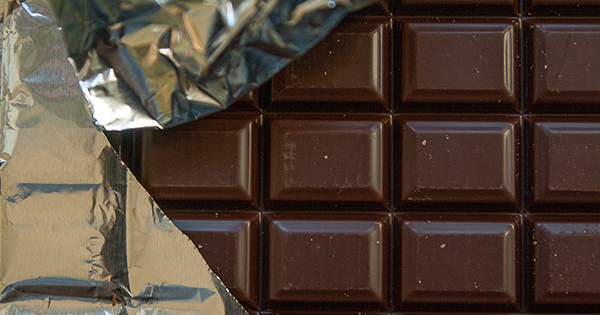# Chocolate

Updated: Oct 26, 2020

Everyone loves chocolate. It tastes good, but it’s also great for demonstrating delicious mathematical ideas like multiplication, division, area and perimeter!Here’s a block. How many pieces are in it?You can count each square individually, but that’s pretty slow. This is where multiplication comes in! The block has 7 rows, each with 8 squares of chocolate, giving 7 x 8 = 56 squares. You could also view it as 8 columns, each with 7 squares, again giving a total 56 squares. 7 x 8 is the same as adding 7 to itself 8 times, or adding 8 to itself 7 times.

How many squares are in half the block?

You can work it out two ways. It’s either 4 rows of 7 = 28, or ½ x 56 = 28.

Similarly a quarter of the block has 2 rows, and 2 x 7 = 14 squares. Or if you prefer, ¼ x 56 = 14.

Sharing = Dividing

You could evenly share the chocolate with 1, 2, 4, 7, 8, 14, 28 or 56 people. These numbers are the factors of 56. What about sharing with 3 people? They could each get 18 squares, but there would be 2 squares left over. This means 56 is not divisible by 3. It’s not the end of the world though – with a little extra work the last 2 squares can be shared equally giving each person 2/3 of a square!

Area and Perimeter

We can also work out the area and perimeter of this block of chocolate. The perimeter is the length around the outside, which is 2 x 7 + 2 x 8 = 30 units. The area, being the 2D space occupied by the block of chocolate, is 8 x 7 = 56 square units.